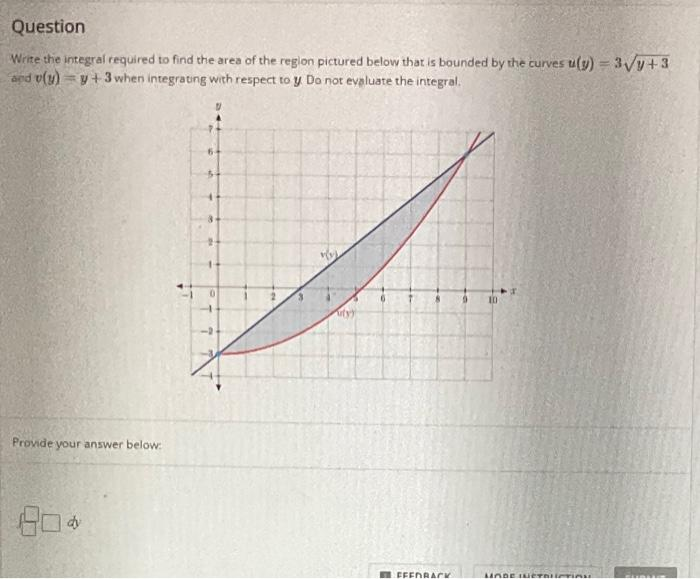# Question Solved1 AnswerQuestion Write the integral required to find the area of the region pictured below that is bounded by the curves (y) = 3V+3 ad v(x) = y + 3 when integrating with respect to y Do not evaluate the integral. 5 1 10 Provide your answer below: EFERAT MADANTranscribed Image Text: Question Write the integral required to find the area of the region pictured below that is bounded by the curves (y) = 3V+3 ad v(x) = y + 3 when integrating with respect to y Do not evaluate the integral. 5 1 10 Provide your answer below: EFERAT MADAN
More
Transcribed Image Text: Question Write the integral required to find the area of the region pictured below that is bounded by the curves (y) = 3V+3 ad v(x) = y + 3 when integrating with respect to y Do not evaluate the integral. 5 1 10 Provide your answer below: EFERAT MADAN